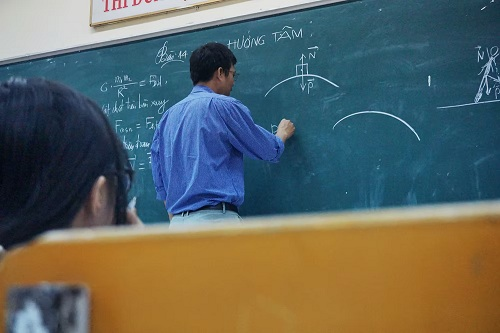# Physics Tips: A Simple Guide To Understanding How Derivatives Work

211

Calculus can be the bane of a student’s existence. The concept, its mathematical notation, and its application can seem foreign to even those who excel at mathematics. But once you understand the basic premise of what a derivative is, how to mathematically express it, and how to use it in applied physics, it will become your best friend in understanding classical (Newtonian) mechanics. While you may need a dense textbook to fully study the field, through this short guide we will help you to grasp the basic concepts. Read on to learn about the various derivative orders and learn how to use them in physics to a model motion under constant acceleration.

#### What Is A Derivative?

In its simplest form, a derivative is a mathematical expression of a rate of change. Any physical action of movement can be expressed by a mathematical equation. This equation will relate the moving object with another object. The rate at which this moving object changes relative to the other object can be expressed as the derivative of that initial mathematical equation. Consider a car travelling down a road. The car is travelling at a certain speed. The car can accelerate, decelerate, or travel at a constant speed. In every scenario, its travel path can be expressed through an equation of that car’s position, which we denote as x, relative to time, which we denote as t. This results in a function; x(t). How fast this position changes relative to time is the derivative; we denote it as x’(t) (read as an ex of tee) or dx/dt. The rate of change of position relative to time is actually just the long way of saying velocity (speed in a certain direction). We can denote velocity as v.

#### Derivative Order

When you have a position as a function of time; x(t), the first-order derivative is the velocity as a function of time; v(t). If we were to examine how fast the velocity changes relative to time, we find the derivative of v(t), which is known as the second-order derivative of x(t). The notation for a second-order derivative can be expressed as x’’(t) or d2x/dt2. This is known as the Acceleration function of time a(t). You can keep going up to higher and higher orders, but as you do that, the physical interpretations become more difficult to comprehend. For example, the rate of change of acceleration relative to time is called a jerk. But rarely will you examine this unit.#### Derivatives And Kinematics

Motion can be studied through kinetics and kinematics. Kinematics focuses on parameters as a function of time whereas kinetics examines motion through forces. They essentially model and examine the same types of motion, but just from a different perspective. There is valuable information in both areas of study, depending on what information you seek. There are four basic equations that examine the motion of a body under constant acceleration; a(t)= c. These equations are:

• v=u+at : Final Velocity = Initial Velocity + Acceleration*Time
• Δx=(0.5uv)+t = Change in Displacement = (0.5*Initial Speed*Final Speed) + Time
• Δx=ut+0.5at2 = Change in Displacement = (Initial Speed * Time) + (0.5*Acceleration*Time*Time)
• V2=U2+2aΔx = Final Speed2 = Initial Speed2 + 2*Acceleration*Change in Displacement

These equations can all be expressed graphically. In a graph of position against time, the gradient of the function expresses the velocity at any given point. In a graph expressing Velocity against time, the gradient represents acceleration. While may be very easy in linear graphs, it is increasingly difficult to evaluate it on graphs with curves. This is where differentiation comes in. Through a mathematical operation (differentiation), you can evaluate the gradient of the curve.

On a graph of x(t) against t, a straight slope means the velocity is constant during that time interval. A perfectly horizontal gradient means that the velocity is 0. This will correlate to the derivative functions which will yield a constant number and a zero, respectively.

The relationships of functions and their derivatives are key to properly modelling physical motion and better understanding the mechanics involved in everyday life. These are principles that our understanding of hasn’t changed in nearly 200 years. To better understand how to differentiate formulas, it is recommended that you have a working knowledge of derivative techniques like the product rule, quotient rule, and chain rule. With that knowledge, with a limited data set, you can understand three different orders of motion.